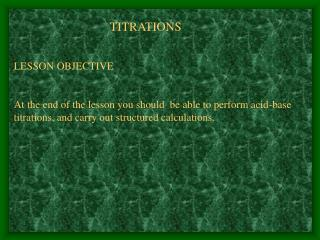DownloadDownload PresentationPerforming acid-base titration

# Performing acid-base titration

Télécharger la présentation## Performing acid-base titration

- - - - - - - - - - - - - - - - - - - - - - - - - - - E N D - - - - - - - - - - - - - - - - - - - - - - - - - - -
##### Presentation Transcript

1. TITRATIONSLESSON OBJECTIVEAt the end of the lesson you should be able to perform acid-base titrations, and carry out structured calculations.

2. An acid-base titration is a volumetric analysis in which a solution of an acid is reacted with a solution of a base.If the concentration of one of the two solutions is known, then using the balanced equation for the reaction the concentration of the other can be deduced.The solution with a known concentration is referred to as a standard solution. The standard solution could be used to determined the following unknown information about the second solution:1. the concentration of the solution2. the molar mass 3. the formula4. the number of molecules of water of crystallisation.

3. Performing acid-base titration • Add a measured volume of one of the solution to a conical flask using a pipette. • The other solution is placed in a burette • The solution in the burette is added to the solution in the conical flask until the end point. • An indicator is used to indicate the end point when it changes colour.

4. An indicator table showing the colour changes

5. Tabulating the results of a titrationResults should be recorded in two decimal places.Do not use the rough figure in your average Use any two or more of the readings which do not differ more than +/- 0.20 for average titre

6. Calculating unknowns from titration resultsIn a titration, 25 cm3 of 0.150 mol/dm3 sodium hydroxide reacted exactly with 23.40 cm3 of sulphuric acid. 2NaOH(aq) + H2SO4(aq)  Na2SO4(aq) + 2H2O(l)(a) Calculate the amount, in mol, of NaOH that reacted.Use n(NaOH) = c x V = 0.150 x 25.0 = 3.75 x 10-3 mol 1000 1000

7. (b)Calculateamount, in mol, of H2SO4(aq)that was used. Equation: 2NaOH(aq) + H2SO4(aq)  Na2SO4(aq) + 2H2O(l) mole ratio: 2 mol 1 mol Actual mole: 3.75 x 10-3 mol 1.875 x 10-3 mol (c) Calculate the concentration in mol/dm3 of the H2SO4(aq) c( H2SO4) = n x 1000 = 1.875 x 10-3 x 1000 = 8.01 x 10-3 mol/dm3 V 23.40

8. 25.00 cm3 of sodium carbonate is neutralised by 27.20 cm3 ofhydrochloric acid of concentration 0.120 mol/dm3. What is the concentration in mol/dm3 of the sodium carbonate solution?(hint: Same method as above )(a) Calculate the amount, in mol, of 27.20 cm3 of HCl (b) Calculate the amount, in mol, of 25 cm3 of Na2CO3(c ) Calculate the concentration in mol/dm3 of the Na2CO3(aq) Answeramount, in mol, of 27.20 cm3 of HCl used = c x V 1000 = 0.120 x 27.20 1000 = 3.264x 10-3mol

9. (b) equation: Na2CO3 + 2HCl  2NaCl + H2O + CO2moles ratio: 1 mol 2 molactual moles: 1.632 x10-3mol 3.264x 10-3mol(c ) Calculate the concentration in mol/dm3 of the Na2CO3(aq) c( Na2CO3) = n x 1000 = 1.632 x 10-3 x 1000 V 25.0 = 6.53 x 10-2 mol/dm3

10. 3. A student dissolved 2.794 g of an acid HX in water and made the solution up to 250 cm3. The student titrated 25.0 cm3 of this solution against 0.0614 mol/dm3 sodium carbonate Na2CO3(aq) were needed to reach the end point. The equation for this reaction is:Na2CO3(aq)+ 2HX(aq)  2NaX(aq) + H2O(l) + CO2(g)(a) calculate the amount, in mol, of Na2CO3 that reacted.(b) Calculate the amount, in mol, of HX that was used in the titration.(c) Calculate the amount, in mol, HX that was used to make up the 250 cm3 solution.(d) calculate the concentration, in g/dm3, of the acid HX.

11. c( Na2CO3) = n x 1000 = 0.0614 x 23.45 V 1000 = 1.44 x 10-3 mol(b) equation: Na2CO3 + 2HX  2NaX + H2O + CO2moles ratio: 1 mol 2 molactual moles: 1.44 x10-3mol 2.88 x 10-3mol(c ) 25.0 cm3 HX(aq) contians 2.88 x 10-3 molso, the 250cm3 solution contains = 10 x 2.88 x 10-3 mol = 2.88 x 10-2 mol(d) from, n = m = 2.794 = 97.0 g/mol M 2.88 x 10-2

12. 5.00 g of an impure sample of potassium carbonate is weighed out and dissolved to make 1.00 dm3 of solution. 25.0 cm3 of this solution is titrated against 0.050 mol/dm3 hydrochloric acid. 28.80 cm3 of the hydrochloric acid is needed to neutralise the potassium carbonate solution.The reaction equation is: K2CO3(aq)+ 2HCl(aq)  2NaCl(aq) + H2O(l) + CO2(g)(a) Calculate the amount, in mol, of 28.80 cm3 HCl that was used in the titration.(b) Calculate the amount, in mol, of 25.0 cm3K2CO3 used.(c) Calculate the concentration, in mol/dm3, of K2CO3 used(d)Deduce the percentage purity of the solid sample of K2CO3

13. ANSWER:(a) amount, in mol, of 28.80 cm3 of HCl used = c x V 1000 = 0.050 x 28.80 = 1.44x 10-3mol 1000(b) equation: K2CO3 + 2HCl  2KCl + H2O + CO2moles ratio: 1 mol 2 molactual moles: 7.20x10-4mol 1. 44x 10-3mol(c ) Calculate the concentration in mol/dm3 of the K2CO3(aq) c( K2CO3) = n x 1000 = 7.20 x 10-4 x 1000 V 25.0 = 2.88 x 10-2 mol/dm3

14. (d) The relative formula mass of potassium carbonate, K2CO3 = ( 2x 39.1) + 12.0 + (3 x 16.0) = 138.2mass of pure K2CO3 in the 2.88 x 10-2 mol/dm3 solution = 2.88 x 10-2 x138.2 = 3.98 g Therefore, this must be the mass of pure potassium carbonate in the original 5.00gpercentage purity = 3.98 x 100 = 79.6 % 5.0

15. Now try questions 1 – 2 Page 29